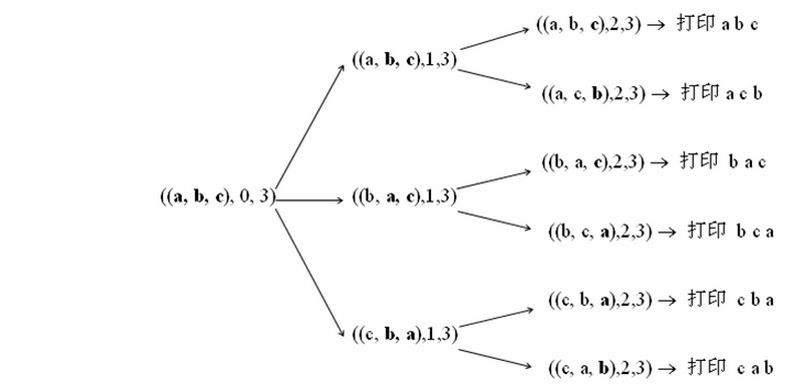﻿ 议事大厅-【赛码网】..
[名企面试知识点][Java]字符串的顺序全排列

## 题目分析

TreeSet 　　　　基于TreeMap实现的SortedSet，可以对Set集合中的元素进行排序，排序后按升序排列元素（缺省是按照自然排序），非线程安全。`import java.util.*;public class Solution {    //用于最后返回结果    ArrayList<String> list  = new ArrayList<>();    //遍历的时候来存储序列实现排序    TreeSet<String> set = new TreeSet<>();    public ArrayList<String> Permutation(String str) {             if(str==null || str.length()==0) return list;       Permutation(str.toCharArray(),0);       //容器转换       list.addAll(set);       return list;    }    public void Permutation(char[] s,int index){        if(s==null ||s.length==0 || index<0 || index>s.length-1)  return ;        if(index==s.length-1){//递归固定到最后一个位置，把该串加入集合            set.add(new String(s));        }else{//固定前index+1个字符，递归后面所有可能的子串           for(int i = index;i<s.length;i++){               swap(s,index,i);//交换一次形成一个子串               Permutation(s,index+1);               swap(s,i,index);//复原使下次循环产生下一个子串           }        }    }    public void swap(char[] s,int i,int j){        char temp = s[i];        s[i] = s[j];        s[j] = temp;    }}`